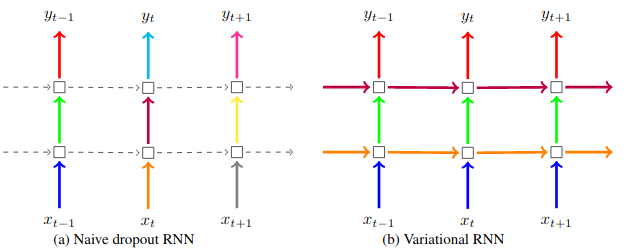# Error : ValueError: can't optimize a non-leaf Tensor

Following, is the code for weightDrop taken from fastAI implementation

``````class WeightDropout(Module):
"A module that warps another layer in which some weights will be replaced by 0 during training."

def __init__(self, module, weight_p, layer_names='weight_hh_l0'):
self.module,self.weight_p,self.layer_names = module,weight_p,L(layer_names)
for layer in self.layer_names:
#Makes a copy of the weights of the selected layers.
w = getattr(self.module, layer)
delattr(self.module, layer)
self.register_parameter(f'{layer}_raw', nn.Parameter(w.data))
setattr(self.module, layer, F.dropout(w.data, p=self.weight_p, training=False))
if isinstance(self.module, (nn.RNNBase, nn.modules.rnn.RNNBase)):
self.module.flatten_parameters = self._do_nothing

def _setweights(self):
"Apply dropout to the raw weights."
for layer in self.layer_names:
raw_w = getattr(self, f'{layer}_raw')
setattr(self.module, layer, F.dropout(raw_w.data, p=self.weight_p, training=self.training))

def forward(self, *args):
self._setweights()
with warnings.catch_warnings():
#To avoid the warning that comes because the weights aren't flattened.
warnings.simplefilter("ignore")
return self.module.forward(*args)

def reset(self):
for layer in self.layer_names:
raw_w = getattr(self, f'{layer}_raw')
setattr(self.module, layer, F.dropout(raw_w.data, p=self.weight_p, training=False))
if hasattr(self.module, 'reset'): self.module.reset()

def _do_nothing(self): pass
``````

I am trying to implement variation dropout: Variational Dropout is dropout which uses same dropout masks at every time step. Pytorch uses dropout in completely AdHoc way as shown in figure (as Naive Dropout) which is wrong and gives unstable results.In variational dropout we should zero out rows of matrices (this is important)so the only change I made is that,

``````def _setweights(self):
"Apply dropout to the raw weights."
for layer in self.layer_names:
raw_w = getattr(self, f'{layer}_raw')

"""
which is the modification that I made for implementing **variation dropout**
"""
N,K  = raw_w.shape